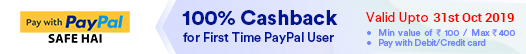Ordinary And Partial Differential Equations: Theory And Applications

By SHAH, NITA H. more
3361 Views
Selling Price : ₹334.13
MRP : ₹495.00
You will save : ₹160.88 after 33% DiscountT&C*
Terms and Conditions
×
• 1. Pay with PayPal and get 100% cashback up to Rs. 400 on your eligible purchases. You will need to transact for a minimum value of Rs. 100 to be eligible for the offer.
• 2. Offer is valid only for first time users/ first ever PayPal transaction in lifetime and can be availed once per PayPal user.
• 3. Offer is valid for transactions through your PayPal India account.
• 4. Cashback will be credited in user’s PayPal account, within 5 days after successful transaction with PayPal.
• 5. Offer period is valid upto 31/10/2019.
• 6. The cashback will be in the form a discount.
• 7. Please see the Terms and Conditions for details.

Save extra with 3 Offers

Get ₹ 50

Instant Cashback on the purchase of ₹ 400 or above

NEW35

Get Flat 35% Off on your First Order

Product Specifications

 Publisher PHI Learning All Engineering Mathematics books by PHI Learning ISBN 9788120350878 Author: SHAH, NITA H. Number of Pages 528 Edition Second Edition Available Available in all digital devicesAbout The Book Ordinary And Partial Differential Equations Book Summary: This revised and updated text, now in its second edition, continues to present the theoretical concepts of methods of solutions of ordinary and partial differential equations. It equips students with the various tools and techniques to model different physical problems using such equations. The book discusses the basic concepts of ordinary and partial differential equations. It contains different methods of solving ordinary differential equations of first order and higher degree. It gives the solution methodology for linear differential equations with constant and variable coefficients and linear differential equations of second order. The text elaborates simultaneous linear differential equations, total differential equations, and partial differential equations along with the series solution of second order linear differential equations. It also covers Bessel’s and Legendre’s equations and functions, and the Laplace transform. Finally, the book revisits partial differential equations to solve the Laplace equation, wave equation and diffusion equation, and discusses the methods to solve partial differential equations using the Fourier transform. A large number of solved examples as well as exercises at the end of chapters help the students comprehend and strengthen the underlying concepts. The book is intended for undergraduate and postgraduate students of Mathematics (B.A./B.Sc., M.A./M.Sc.), and undergraduate students of all branches of engineering (B.E./B.Tech.), as part of their course in Engineering Mathematics. New to the SECOND Edition • Includes new sections and subsections such as applications of differential equations, special substitution (Lagrange and Riccati), solutions of non-linear equations which are exact, method of variation of parameters for linear equations of order higher than two, and method of undetermined coefficients • Incorporates several worked-out examples and exercises with their answers • Contains a new Chapter 19 on ‘Z-Transforms and its Applications’. Table of Contents: Preface 1. Introduction of Ordinary Differential Equation 2. Differential Equations of the First Order and First Degree 3. Differential Equations of First Order and of Higher Degree 4. Linear Differential Equations with Constant Coefficients 5. Homogeneous Linear Differential Equations with Variable Coefficients 6. Exact Differential Equations and Differential Equations of Higher Order 7. Linear Differential Equations of Second Order 8. Simultaneous Linear Differential Equations 9. Total Differential Equations 10. Partial Differential Equations (PDE) of First Order 11. Linear Partial Differential Equations with Constant Coefficients 12. Partial Differential Equations of Order Two with Variable Coefficients 13. Power Series Method 14. Bessel’s Equation and Bessel’s Function 15. Legendre’s Equation and its Polynomials 16. Laplace Transform and its Applications 17. Applications of Partial Differential Equations of Order Two 18. Fourier Transforms and its Applications to Partial Differential Equations 19. Z-Transforms and its Applications Index

Related Engineering Mathematics Books
x